# Category cohomology

(diff) ← Older revision | Latest revision (diff) | Newer revision → (diff)

Letbe a small category,an Abelian category with exact infinite products, and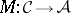a covariant functor. Define the objects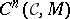for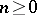in the following way: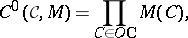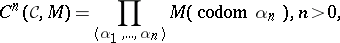where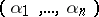is a sequence of morphisms ofwith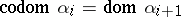,. Let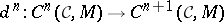be the monomorphism induced by the family of morphisms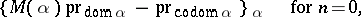and by the family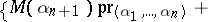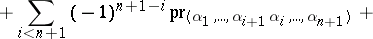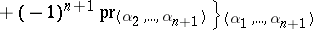Here,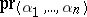denotes the projection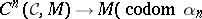).

The morphisms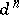,satisfy the conditions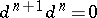, and therefore one obtains a complex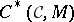in. The homology objects of this complex are called the cohomology of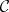with coefficients inand are denoted by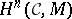. For any functorthere are a functor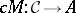(called the co-induced functor) and a monomorphism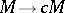such that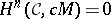for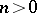.

The functor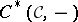is an exact functor. Therefore, any short exact sequence of functors induces a long exact sequence of cohomology objects of. It can be proved that the functors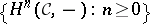form a universal connected (exact) sequence of functors and that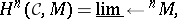whereisth right satellite of the functor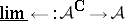(here,denotes the category of functors fromto, and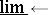is an (inverse) limit functor).

For a small category, letdenote the pre-additive category whose objects are those ofandis the free Abelian group on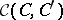(cf. also Free group). Composition is defined in the unique way so as to be bilinear and to make the inclusion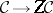a functor. Ifis a monoid, then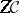is the monoid ring ofwith coefficients in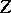. The inclusioninduces an isomorphism of categories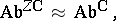where the left-side is the category of additive functors fromto(the category of Abelian groups) and the right-hand side side is the category of all functors fromto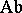.

Ifis the category of Abelian groups (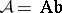), one haswhere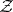denotes the constant functor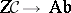(i.e.,for any objectand any morphismof), andis taken in the category of additive functors.

Ifis a group (i.e. a category with one object and whose morphisms are invertible) and, then the groupsare the cohomology groups (cf. also Cohomology group) of the groupwith coefficients in, which is a module over the group ring(cf. also Cross product). In this case the co-induced functoris a co-induced-module.

As for the case of groups,-fold extensions of categories can be defined and the isomorphism withcohomologies of categories can be established. Under additional assumptions, the properties of group cohomologies are obtained for category cohomologies (e.g. the universal coefficient formula for the cohomology group of a category, etc.).

For a commutative ring, a-category is a categoryequipped with a-module structure on each hom-set in such a way that composition induces a-module homomorphism. If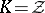, a-category is just a pre-additive category. B. Mitchell has defined the (Hochshild) cohomology group of a small-categorywith coefficients in a bimodule (i.e., bifunctor)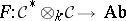(where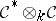denotes the tensor product of categories). H.-J. Baues and G. Wirshing have introduced cohomology of a small category with coefficients in a natural system, which generalizes known concepts and uses Abelian-group-valued functors (i.e. modules) and bifunctors as coefficients.

How to Cite This Entry:
Category cohomology. Encyclopedia of Mathematics. URL: http://encyclopediaofmath.org/index.php?title=Category_cohomology&oldid=12906
This article was adapted from an original article by T. Datuashvili (originator), which appeared in Encyclopedia of Mathematics - ISBN 1402006098. See original article